NCERT Solutions for Class 6 Maths Chapter 13 Symmetry Ex 13.1 are part of NCERT Solutions for Class 6 Maths. Here we have given NCERT Solutions for Class 6 Maths Chapter 13 Symmetry Ex 13.1.

 Board CBSE Textbook NCERT Class Class 6 Subject Maths Chapter Chapter 13 Chapter Name Symmetry Exercise Ex 13.1 Number of Questions Solved 6 Category NCERT Solutions

## NCERT Solutions for Class 6 Maths Chapter 13 Symmetry Ex 13.1

Question 1.
List any four symmetrical objects from your home or school.
Solution :
The blackboard, the table top, a pair of scissors, the computer disc.

Question 2.
For the given figure, which one is the mirror line, l1 or l2?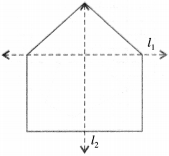Solution :
I2 is the mirror line.

Question 3.
Identify the shapes given below. Check whether they are symmetric or not. Draw the line of symmetry as well.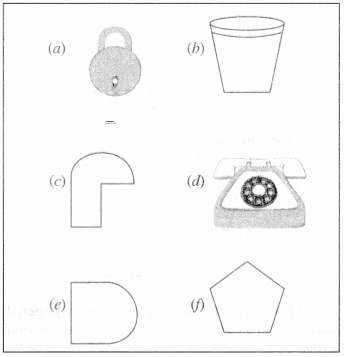Solution :
(a) Symmetric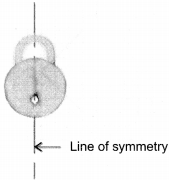(b) Symmetric(c) Not symmetric
(d) Symmetric(e) Symmetric(f) Symmetric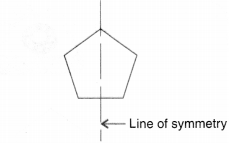Question 4.
Copy the following on a squared paper. A square paper is what you would have used in your arithmetic notebook in earlier classes. Then complete them such that the dotted line is the line of symmetry.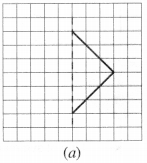Solution :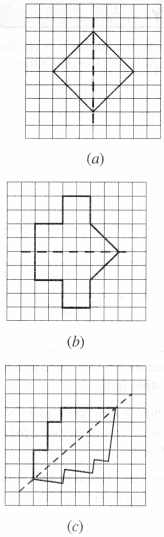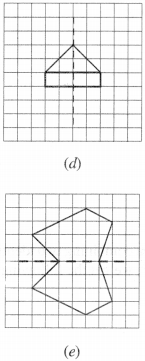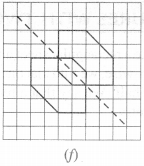Question 5.
In the figure, l is the line of symmetry. Complete the diagram to make it symmetric.Solution :Question 6.
In the figure, l is the line of symmetry. Draw the image of the triangle and complete the diagram so that it becomes symmetric.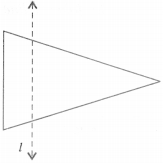Solution :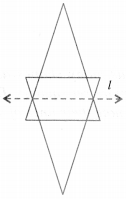We hope the NCERT Solutions for Class 6 Maths Chapter 13 Symmetry Ex 13.1 help you. If you have any query regarding NCERT Solutions for Class 6 Maths Chapter 13 Symmetry Ex 13.1, drop a comment below and we will get back to you at the earliest.